# MCAT Chem/Phys Math Strategies From a 98th Percentile Scorer

February 21, 2023

Get more top scorer strategies like this in the Chem/Phys & Bio/Biochem Mastery Course!

MCAT Chem/Phys math calculations can sometimes feel very overwhelming!

Even a lot of your highest scoring mentors here have struggled on practice exams with remembering equations, not feeling confident about the equation needed, or being forced to guess and move on from these questions on the exam so we don’t burn through our test time.

If you can relate to any of those difficulties or if you just want quicker ways to get to the correct answer without knowing (or memorizing) equations, we have a treat for you…

First, a few things to keep in mind.

1. Most calculation questions do require some form of an equation, but don’t rely on this as the main form of calculating. It’s helpful though if you struggle with remembering equations and remembering them all correctly since you’ll be able to remember without doing a great deal of work and memorization.
2. Know the units you’re working with and the SI units (the metric system). This means, for example, not just knowing that work is a unit of joules but also knowing what actually is a joule. And it can be overwhelming to see all the kilograms, meters, and seconds for all the SI units but it’ll go a long way, and they’re all interconnected and very helpful. Think: how are equations and variables related?
3. A lot of MCAT chem/phys math equations rely on basic conceptualizations and equations so know the concept behind what the equation is showing instead of straight-up memorizing an equation at face value. If you don’t understand the concept, you won’t be able to correctly answer the most basic questions because they’re testing your basic conceptual knowledge, not how you really memorized the equations.

Knowing these things will generally help you do better on MCAT chem/phys math, but let’s now dive into different AAMC practice questions for specific examples of these strategies…

## MCAT Chem/Phys Math Example #1: Section Bank Passage 1 Question 1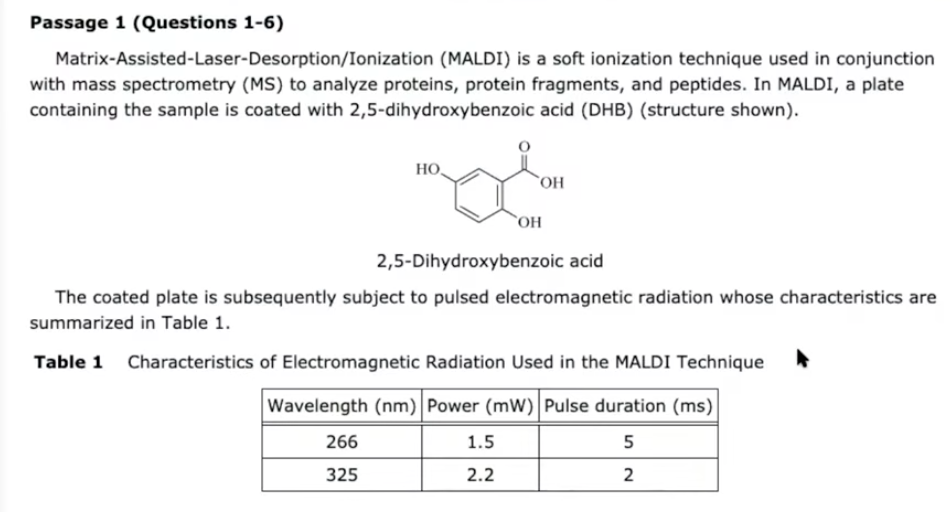This passage is all about a technique called MALDI that is used with mass spectrometry to analyze proteins, protein fragments, and peptides.

In Table 1 above, they give you the characteristics of the radiation that’s used in this technique…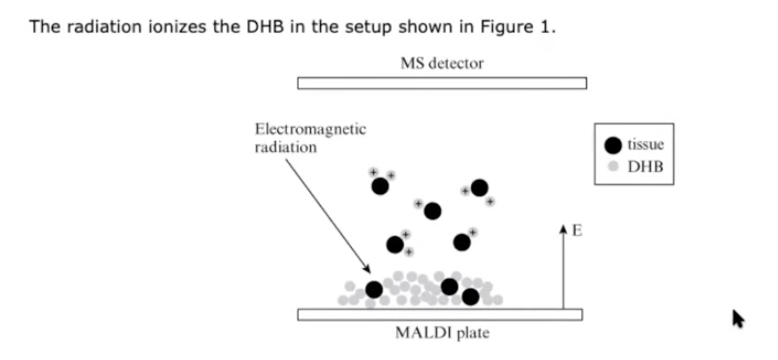And they give you a diagram of the whole set-up.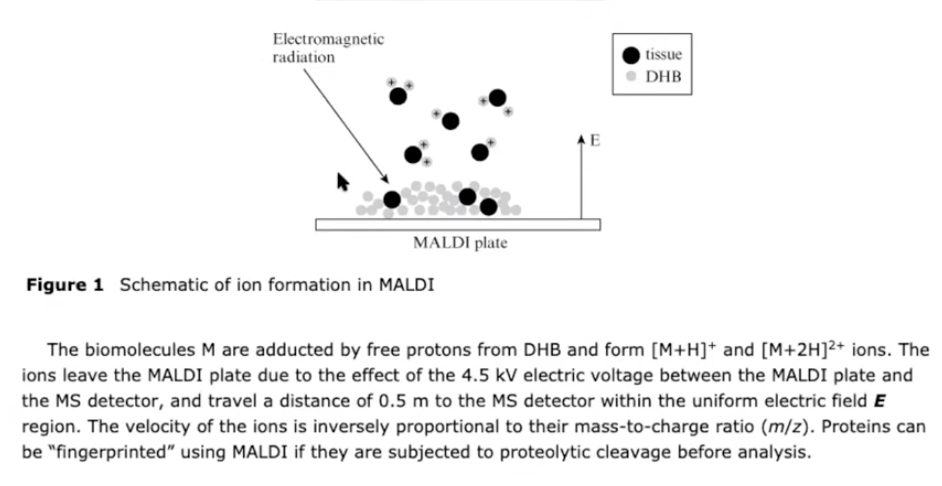They then explain that this electronic voltage between the MALDI plates and the detector is what allows these ions to move upwards towards the detector. They say the distance that it’s traveled, that there’s a uniform electric field, and how to calculate the velocity. They do mention that it can be fingerprinted as long as they’re cleaved via proteolysis before analysis.

Question:

What is the magnitude of the electric field used in the MALDI-MS imaging device described in the passage?

1. 1 kV/m
2. 3 kV/m
3. 6 kV/m
4. 9 kV/m

I know that electric field and electric voltage—these things can be overwhelming and if you don’t know one of the equations for electric fields (because there are a couple), this is one of the questions where you don’t need it. Luckily, all the answer choices are in the same unit. This means that you know that the answer choice is in kilovolts per meters.

Sometimes, the answer choice is in a different unit and, if that’s the case, if you don’t know how to go about it then you most likely won’t be able to use this method. It only works if you know what the units have to be for the answer.

So knowing that we’re looking for kilovolts and we’re looking for meters. My eye directly goes into the center of the last paragraph where it’s talking about kilovolts and meters. You take 4.5 kilovolts and you divide it by .5 meters, and that gives you an answer of 9 kV/m.

Equation we used:

E=v/m

If you’re early in your MCAT chem/phys math and content prep or just unsure of what content will be covered in this section, then we recommend you check out Kaplan’s content guideline for chem/phys!

## MCAT Chem/Phys Math Example #2:  Section Bank Passage 1 Question 5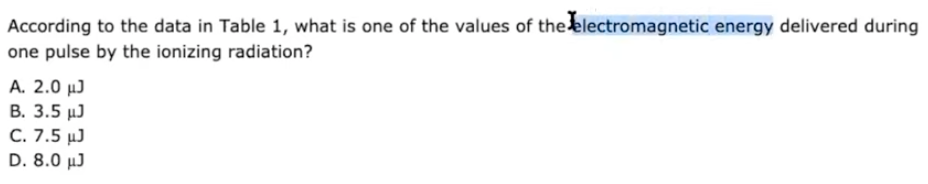We know that we’re looking for electronic magnetic energy and that we’re using Table 1. So in Table 1, we’re given wavelength, power, and pulse duration.

We also know that our units are in joules and you should be familiar with the unit of joules. You should know that this is a unit that is for work and for energy, which makes sense because we’re talking about electromagnetic energy.

Now you might be a little bit confused because in Table 1 you don’t see any work there, you don’t see any energy there. You just see the two lasers with their wavelengths of 266 and 325 and their properties of power and the duration.

However, we should know how power and work are related on a conceptual level. There’s a very simple equation that goes along with it, but it’s really more about the basic concept and you’d understand the concept especially if you know the SI base units (the metric system) for power and for work because:

The SI (metric system) unit for power is the same for work except an additional second is added onto the denominator:

W/seconds = P

That is:

Power = Work/Time

We can use this very basic conceptual knowledge to answer this question which is giving you power and giving you seconds. And if we multiply these two, then we will end up getting an answer of work or energy which would therefore be in joules.

So for this question, we multiply both of them because we’re not sure which one is actually the correct answer.

1.5 x 5 = 7.5

2.2 x 2 = 4.4

So we have our first answer, 7.5. There is no 4.4 and there can’t be because you’d have two correct answers and that’s not possible.

## MCAT Chem/Phys Math Example #3: Chemistry Question Pack Question 81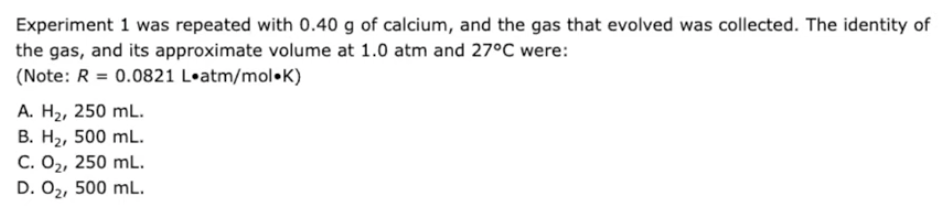They’re asking you what this gas is and how many milliliters  (volume) were collected.

I’m going to focus on the latter portion of these answer choices which is calculation.

We’re very lucky because our answer choices are in liters, so we know our answer should be in liters of some sort, then we’ll convert it to mL accordingly.

Looking at what they give you in the question stem, which is so important, we have the R value; we see the units are in L x atm divided by mol x Kelvin. This liter is what we’re looking for—so that’s my first thought, think how do I isolate that liter by getting rid of some of these other units? And there are quite a few numbers given to us that we can use.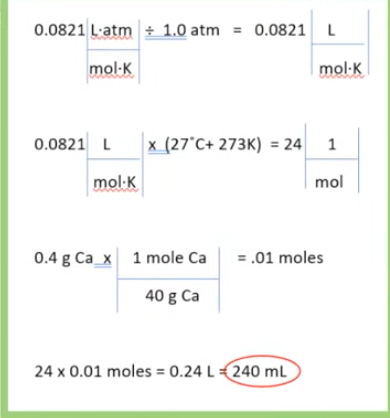We’re lucky that 250 is very close to our answer of 240mL so we know here that the answer choice has to be A or C. Now we can cross out B and D, and the rest is conceptual based on the passage and at least we have our answer choice narrowed down to 2.

We figured out the calculation!

If you’re finding this passage dissection helpful so far, you should also check out our article on the top strategies that will raise your MCAT chem/phys skills and section score fast here!

## MCAT Chem/Phys Math Example #4: Physics Question Pack Question 45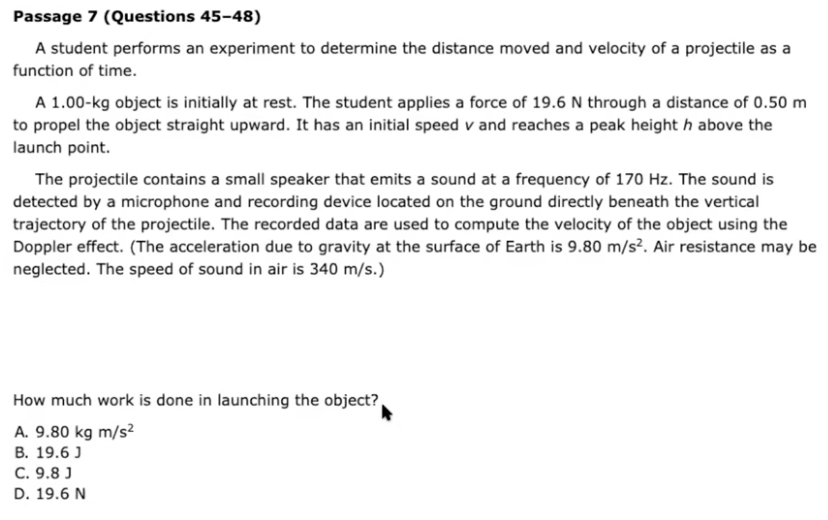This is where your knowledge of the SI units (the metric system) comes in handy.

With work, we know that the unit is in joules.

Looking at the answer choices quickly, we can immediately cross out D because work is not Newtons, that is force and not work at all.

Now, looking at A, this might be tricky if you don’t know your SI base units. However, if you do, you know that work is not that. So we can also cross out A.

Moving on to B and C. Even if we don’t know the equation and have no idea how to do this, we can reason about it a little bit.

Because we have units of 19.6 and I immediately look at the second paragraph because 19.6 is mentioned twice. This is the force they’re talking about and, if I’m on my last resort, my guess would be that the work is going to be at a value that’s different from the initial force. But this is not always the case…

Sometimes the answer might be based on something in the passage but with the MCAT chem/phys math, things don’t usually stay the same. In this case, there’s an object that is moving. Usually, the answer would be different, especially if we’re talking about force and Newtons, we’re trying to get a change of energy or work in joules. So my best guess would be C because we know that it’s joules or change of energy and we know that it’s different. And that’s the correct answer.

This might be a little more of a guesswork. If you knew the equation, you’d be able to figure this out that way.

## MCAT Chem/Phys Math Example #5: AAMC Practice Exam C/P Section Question 39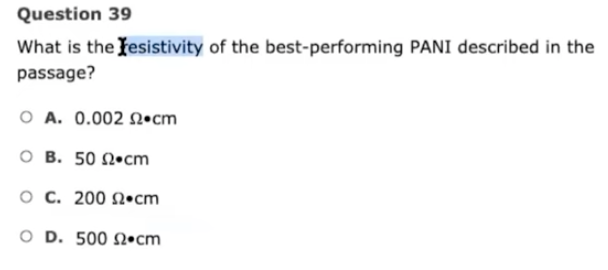This is a question about resistivity, and it’s a very simple question that you should be familiar with but it doesn’t come up that often.

So, we know that our units are in ohms x centimeter, so we know this has to be the answer of our answer choice.

The only time we see ohms in the passage is in the last paragraph where it says that the best-performing PANI had a maximum conductivity of [...] so we know that this is actually on the denominator, there’s only a number 1 on the numerator. So I immediately think, these are my units, but inverted. So in order to get to the units I need, I have to flip it.

The hardest part about this isn’t understanding that, it’s understanding how to invert a decimal. The inverse of  0.005 is 200, so that is the answer choice C.

## MCAT Chem/Phys Math Example #6: AAMC Practice Exam C/P Section Question 50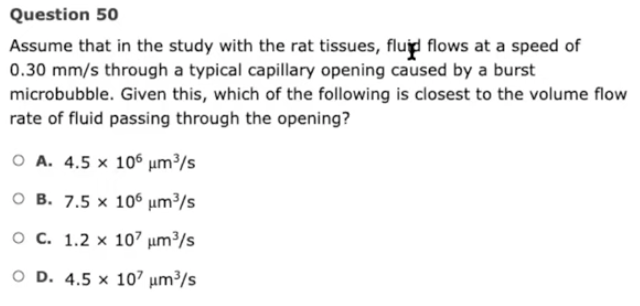Again, the units are all the same so we know they have to be in µm3/second.

I know that I’m given mm/second in the passage so I’m thinking I’m going to be using this at some point, and this will give me the seconds that are in my unit. We also have meters, but meters cubed. So we think we might need to multiply it by meters twice, so m x m x m; or multiply this by a meter squared, which would also get me to µm3.

Looking through the passage, we have a range of diameters mentioned here, which are both in meters, but the fact that it’s a range is a little bit confusing because which one would we choose. In the end, we see µm² and we like that because we have that and if we multiply it by regular meters, we end up with µm3, and the mm/seconds will give me my seconds and that will be my answer choice.

Let’s multiply 0.3 x 2.5 and figure out the prefix based on the two that are given.

That gives us an answer of 0.75 so I know my answer choice will be something with a 7 and a 5. I don’t know exactly how it’s going to work out once I figure this out, but we’re lucky because the answer choice, we don’t need to work with the micro and the milli to figure out how to convert it into micro. Just stick to the fact that there’s going to be 0.75 or 7.5 or 750,000, whatever it is. And you know that you have a 7.5 in answer choice B.

## MCAT Chem/Phys Math Example #7: AAMC Practice Exam C/P Section Question 59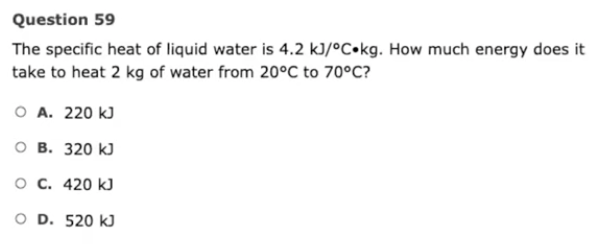This comes off as confusing at first because everytime there is “specific heat” we might think oh my god what’s the equation because there’s quite a couple. All you need is a simple analysis.

All your answers are in kJ so you’re looking for kJ or joules as your final answer. We see kJ given in this initial unit given to us and, if we have to isolate kJ, we have to somehow get rid of celsius and kilograms.

To get rid of our kilograms:

4.2 x 2 = 8.4

To get rid of celsius:

70 - 20 = 50°C

Thus:

50°C x 8.4 = 420

And that’s it!!

We hope you found the video and commentary helpful and have realized how you really can use basic concepts, unit knowledge and the multiple choice options to help you find correct answers even in situations where you’re not very confident with the equations you’re using.

Remember:

• Know your units (in SI/metric system)
• Know the basic concepts behind them and how they’re related

If you found Aly’s video and passage dissection helpful and are still looking for ways to improve your chem/phys math and section score, then you should definitely check out our chem/phys + bio/biochem strategy course and complete MCAT strategy course!

Our top scoring mentors, like Aly, have put together their best MCAT strategies and approaches (including many more passage dissections like in this video!) all in one place so that you can get through these challenging sections with as little difficulty as possible.

Long passages and questions like the ones you’ll see on the chem/phys section of the MCAT can sometimes really test your patience and commitment during your MCAT prep…

Just remember that you can and will overcome these challenges and move one step closer to becoming a doctor!

The MCAT Mastery Team
We're a team of future doctors passionate about giving back and mentoring other future doctors! All mentors on the team are top MCAT scorers and we all are committed to seeing you succeed in achieving your physician dreamsTo help you achieve your MCAT score to the competitive mark quickly, we have collaboratively created these self-paced MCAT strategy courses and are also available for one-on-one tutoring!